## Calculating the price of a bond with an odd last coupon in SQL Server

Nov 24

Written by: Charles Flock
11/24/2014 1:11 PMBonds with an odd last coupon will arise infrequently in any portfolio. With XLeratorDB you can be confident that when they do show up in your portfolio you will be able to do all the necessary calculations just as easily as you can for bonds that pay regular periodic interest.
We have previously written about calculating price, yield, and accrued interest for bonds paying regular periodic interest as well as for bonds with an odd first coupon period. In this article we look at the price, yield and accrued interest calculations for bonds with an odd last coupon period and compare the XLeratorDB functions to the Excel functions.
A bond has on odd last coupon (OLC) when the last coupon period is either longer or shorter than all the other coupon periods which otherwise occur at regular intervals. Unlike off first coupon (OFC) bonds (discussed here), the OLC formula is used for every settlement date. Because of this there are actually 2 OFC formulas; the first for settlement prior to the last coupon date and the second for when settlement occurs on or after the last coupon date. When settlement occurs prior to the last coupon date, the formula is: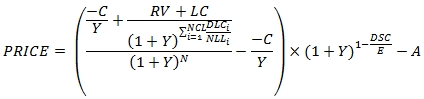Where

 C = 100 * coupon rate / frequency Y = yield / frequency RV = redemption value DSC = number of days from settlement to coupon N = the number of coupons between the settlement date and the last coupon date E = the number of days in the current coupon period A = C * accrued days / E NCL = the number of quasi-coupons from the last coupon date to the quasi-maturity date DLCi = the number of days from the previous coupon date to the lesser of the next coupon date and the maturity date in the ith quasi-coupon period NLLi = the normal length in days of the full ith quasi-coupon period in the odd last period LC = C *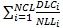This formula is very similar to the formula for bonds that pay regular periodic interest (RPI) with the exception of one term: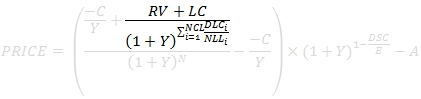In the RPI formula, this term is simply the redemption value (RV). In this formula, two adjustments are made to RV.
First, the last coupon amount (LC) is added to the redemption value. The last coupon is calculated using the fraction of the number of days from the last coupon date to the maturity date (DLC) and dividing it by the normal length (NLL) of the coupon period.
This requires determining if the last coupon period is a long period (meaning that it is longer than normal) or a short period. We do this by calculating a quasi-maturity date, moving forward from the last coupon date until the quasi-maturity date is greater than or equal to the actual maturity date. This starts with the last coupon date and advances by 12/Frequency months until the maturity date is greater than or equal to the settlement date. If the last coupon date is the last day of the month, then the quasi-maturity is influenced by the basis code. For example, if the last coupon date is 2014-06-30 and the frequency is 2 (meaning the coupons are paid twice-yearly) and the day-count convention is Actual/Actual then the quasi-maturity date is calculated as 2014-12-31. If the day-count convention was Actual/Actual Non-EOM instead, then the quasi-maturity date is 2014-12-30.
We then calculate the DLC/NLL ratio for each quasi-coupon period and add them up. This factor is then used to calculate the last coupon amount.
Second, using the same factor, the RV + LC amount is discounted back to the last coupon date.
Having done that, we can then simply use the RPI calculation to calculate the price of the bond. One thing that you should take note of, however, is that the Excel ODDFPRICE calculation does not support this, as it requires the settlement date to be greater than the last coupon date. Let's look at an example of this.

 Settlement: 2014-11-25 Maturity: 2034-11-01 Last Coupon 2034-06-15 Basis Bond Coupon Rate 2.65% Yield 2.95% Redemption Value 100 Coupon Frequency 2

In XLeratorDB we can simply enter the following statement:
SELECT
wct.ODDLPRICE(
'2014-11-25' --@Settlement
,'2034-11-01' --@Maturity
,'2034-06-15' --@LastCoupon
,0.0265       --@Rate
,0.0295       --@Yield
,100          --@Redemption
,2            --@Frequency
,0            --@Basis
) as PRICE
This produces the following result.
PRICE
----------------------
95.5031825457956
When we enter the same data into Excel it returns #NUM!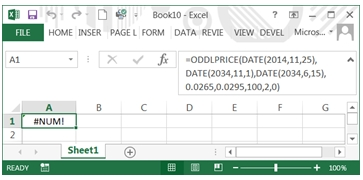This is actually consistent with the Excel documentation:
"The following date condition must be satisfied; otherwise, ODDLPRICE returns the #NUM! error value:
maturity > settlement > last_interest"
I completely understand why you would want the maturity date to be greater than the settlement date, but why is it a requirement that settlement be greater than last_interest? Do these bonds not have a price prior to the last coupon date? That's nonsensical.
Maybe it's possible that we are not understanding the Excel documentation. For example, when I refer to the last coupon date, I am referring to the date of the last regular periodic coupon. This date is then the commencement of the odd last coupon date which terminates on the maturity date when the redemption value and the odd coupon interest are paid out. How does Excel define the last coupon date, which they refer to as Last _interest?
“The security's last coupon date. “
That's helpful. Maybe they mean the most recent coupon date prior to the settlement date? But, as explored in great detail here, that doesn't make any sense either.
Usually when I have a problem with an Excel function I explore the same function in Google spreadsheets. However, Google spreadsheets does not have an ODDLPRICE function.
Since Excel does not support the pricing of an odd last coupon bond until the settlement date is greater than the last coupon date it also does not support the calculation of the yield unless the settlement date is greater than that last coupon date. I would think that this pretty much rules out the Excel ODDLPRICE and ODDLYIELD functions under any circumstances. But let's look at the math behind the calculation when the settlement date is in the last coupon period. Note that when the settlement date is equal to the last coupon date it is in the last coupon period even though Excel will return #NUM!
The OLC formula for a bond settling on or after the last coupon date is: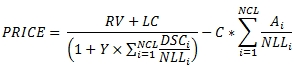Where

 C = 100 * coupon rate / frequency Y = yield / frequency RV = redemption value NCL = the number of quasi-coupons from the last coupon date to the quasi-maturity date DSCi = number of days from settlement date (or beginning of quasi-coupon period) to the next quasi-coupon within odd period (or to redemption date) for the ith quasi-coupon period Ai = number of accrued days for the ith quasi-coupon period within odd period counting forward from the last interest date before redemption DLCi = the number of days from the previous coupon date to the lesser of the next coupon date and the maturity date in the ith quasi-coupon period NLLi = the normal length in days of the full ith quasi-coupon period in the odd last period LC = C *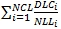The function call for settlement in the last coupon period is exactly the same as in the previous example; we are just calling the function with different data and it makes the determination of which formula to use.
SELECT
wct.ODDLPRICE(
'2014-11-17' --@Settlement
,'2015-01-15' --@Maturity
,'2014-09-01' --@LastCoupon
,0.0500       --@Rate
,0.0030       --@Yield
,100          --@Redemption
,2            --@Frequency
,1            --@Basis
) as PRICE
This produces the following result.
PRICE
----------------------
100.765127973736
And we can see that Excel returns the same result.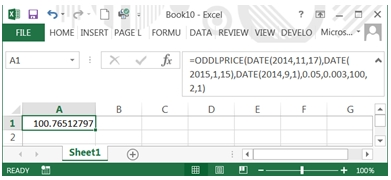One of the bigger challenges we have discovered in benchmarking XLeratorDB against Excel, is that it is extremely difficult to discern how Excel determines the inputs into an equation. In the case of ODDLPRICE, with settlement in the final coupon period, the formula contains a handful of variables which are calculated by the function, but is otherwise mathematically straightforward. Thus we decided to provide a function, OLCFACTORS, which enumerates the values used in the calculation. The call to the function is almost the same as the call to the ODDLPRICE function, except that you have the option of using either the price or the yield in the function call.
SELECT
*
FROM
wct.OLCFACTORS(
'2014-11-25' --@Settlement
,'2034-11-01' --@Maturity
,'2034-06-15' --@LastCoupon
,0.0265       --@Rate
,NULL         --@Price
,0.0295       --@Yield
,100          --@Redemption
,2            --@Frequency
,0            --@Basis
)
This produces the following result which I have reformatted for ease of viewing.

 A NULL DSC NULL E NULL N 0 NCL 1 A1 77 DSC1 59 DLC1 136 NLL1 181 A2 NULL DSC2 NULL DLC2 NULL NLL2 NULL quasicoup NULL quasimaturity 1-Mar-15 C 2.5 LC 1.878453039 P 100.765128 AI 1.063535912 Y 0.003

If we wanted to, we could use these values and construct our own calculation of ODDLPRICE in a SELECT statement.
SELECT
(100 + LC)/(1+.003/2*DSC1/NLL1)-AI as PRICE
FROM
wct.OLCFACTORS(
'2014-11-17' --@Settlement
,'2015-01-15' --@Maturity
,'2014-09-01' --@LastCoupon
,0.0500       --@Rate
,NULL         --@Price
,0.0030       --@Yield
,100          --@Redemption
,2            --@Frequency
,1            --@Basis
)
This produces the following result.
PRICE
----------------------
100.765127973736
We liked that idea so much, that we created a generic function, OLC, which will let you calculate the price or yield of the odd last coupon bond using just the variables, making the calculation very explicit. For this example we could have entered the following SQL to calculate the price.
SELECT
wct.OLC(
.05          --@Rate
,.003         --@Yield
,NULL         --@Price
,100          --@Redemption
,2            --@Frequency
,NULL         --@A
,NULL         --@E
,NULL         --@DSC
,0            --@N
,'True'       --@ShortLast
,77           --@A1
,59           --@DSC1
,136          --@DLC1
,181          --@NLL1
,NULL         --@A2
,NULL         --@DSC2
,NULL         --@DLC2
,NULL         --@NLL2
) as PRICE
This produces the following result.
PRICE
----------------------
100.765127973736
We found this function very useful even in calculations where the settlement date was greater than the last coupon date because the Excel result left us scratching our heads a little bit. For example,
SELECT
wct.ODDLPRICE(
'2014-11-17' --@Settlement
,'2014-12-15' --@Maturity
,'2014-06-30' --@LastCoupon
,0.0500       --@Rate
,0.0100       --@Yield
,100          --@Redemption
,2            --@Frequency
,1            --@Basis
) as PRICE
returns the following result in XLeratorDB.
PRICE
----------------------
100.302670227285
But, in Excel, we get a different result.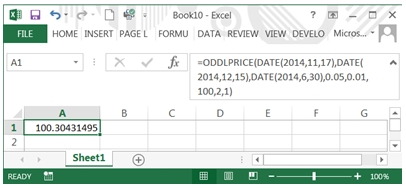If you are wondering what causes the difference, EXCEL calculates the quasi-maturity date as 2014-12-30 (an aspect of the calculation that is completely undocumented) and XLeratorDB calculates the quasi-maturity date as 2014-12-31 (because the basis code is 1; if it were 11 it would use 2014-12-30, but Excel does not provide that flexibility).
SELECT
wct.ODDLPRICE(
'2014-11-17' --@Settlement
,'2014-12-15' --@Maturity
,'2014-06-30' --@LastCoupon
,0.0500       --@Rate
,0.0100       --@Yield
,100          --@Redemption
,2            --@Frequency
,11           --@Basis
) as PRICE
This produces the following result.
PRICE
----------------------
100.304314952698
In fact, XLeratorDB supports a range of features and capabilities in ODDLPRICE which are not supported in EXCEL.
·         Settlement any time prior to the maturity date (Excel only supports settlement after the last coupon date)
·         Monthly and bi-monthly frequencies
·         Negative coupon rates
·         Negative yields
·         More day-count conventions including 30E+/360, NL/360, NL/365, NL/Actual, and Actual/364
·         Support for non-end-of-month date calculations for all day-count conventions
In terms of calculating the yield for these type of bonds, Excel has exactly the same issues in ODDLYIELD as it does in ODDLPRICE. Whereas SQL Server users using XLeratorDB can enter
SELECT
wct.ODDLYIELD(
'2014-11-25' --@Settlement
,'2034-11-01' --@Maturity
,'2034-06-15' --@LastCoupon
,0.0265       --@Rate
,95.503183    --@Price
,100          --@Redemption
,2            --@Frequency
,0            --@Basis
) as YIELD
and get the following result;
YIELD
----------------------
0.0294999996884782
this is what happens in Excel.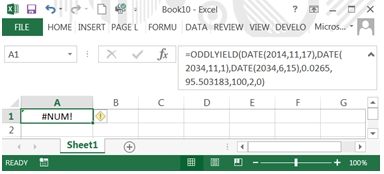Of course, this is because like the Excel ODDLPRICE function, the Excel ODDLYIELD function requires that the settlement date be greater than the last coupon date.
Finally, let us turn to the issue of accrued interest. We have previously written about the problems with the Excel ACCRINT function (here and here) and you should probably avoid using it. Let's just look at a simple example.

We have implemented a function, ODDLINT, specifically for the calculation of the accrued interest in the last coupon period because it is different than calculating the accrued interest in the odd first coupon period and the accrued interest during a regular coupon period. In this example we are calculating the accrued interest in the odd last coupon period for a bond with a maturity date of 2015-01-15 a last coupon date of 2014-06-30 and a settlement date of 2014-12-28.
SELECT
wct.ODDLINT(
'2014-12-28' --@Settlement
,'2015-01-15' --@Maturity
,'2014-06-30' --@LastCoupon
,0.05         --@Rate
,2            --@Frequency
,1            --@Basis
) as [AI]
This produces the following result.
AI
----------------------
2.45923913043478
Let's try to fit the same calculation into the ACCRINT function in Excel.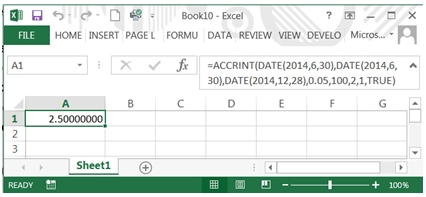We don't even need to do much math to see that the calculation is wrong. A full coupon payment is 2.5. We asked Excel's ACCRINT function to calculate the interest from 2014-06-30 (the previous coupon date) and 2014-12-28 (the settlement date) and it returned the full coupon payment. Whether or not you think that a full coupon period runs from the 30th of June to the 30th of December or from the end of June to the end of December, it clearly does not run from the 30th of June to the 28th of December. It is best to avoid using the ACCRINT function in Excel.
You can also use the XLeratorDB table-valued function OLCFACTORS to get the accrued interest regardless of the settlement date as well as the AIFACTOR function. Here's an example where we calculate the accrued interest on the day before the last coupon date, the day of the last coupon, and the day after the last coupon.
SELECT
n.settlement
,k.AI
,wct.AIFACTOR(
1             --@Basis
,.05          --@Rate
,'2015-01-15' --@Maturity
,n.settlement --@Settlement
,2            --@Frequency
,NULL         --@FirstCouponDate
,'2014-06-30' --@LastCouponDate
,NULL         --@IssueDate
,NULL         --@Holidays
) * 100 as [AIF]
FROM
(VALUES
('2014-06-29')
,('2014-06-30')
,('2014-07-01')
)n(settlement)
CROSS APPLY
wct.OLCFACTORS(
n.settlement --@Settlement
,'2015-01-15' --@Maturity
,'2014-06-30' --@LastCoupon
,0.0500       --@Rate
,NULL         --@Price
,0.0030       --@Yield
,100          --@Redemption
,2            --@Frequency
,1            --@Basis
)k
This produces the following result.
settlement AI                     AIF
---------- ---------------------- ----------------------
2014-06-29 2.48618784530387       2.48618784530387
2014-06-30 0                      0
2014-07-01 0.0135869565217391     0.0135869565217391

XLeratorDB provides a highly-optimized, efficient, scalable solution for dealing with all types of bond calculations, many of which are not supported in Excel. By using SQL Server with XLeratorDB as the platform for your bond calculations you can take advantage of the world's most flexible and widely used tool for data management; SQL.

Download the free 15-day trial and discover how easy it is to get going.

Tags:
Categories:
Location: BlogsThe WestClinTech Blog

## Search Blogs

 KeywordsPhrase

## Blog Archives

Archive
 < September 2023 >
SunMonTueWedThuFriSat
272829303112
3456789
10111213141516
17181920212223
24252627282930
1234567
Monthly
Go

### SupportCopyright 2008-2023 Westclintech LLC         Privacy Policy        Terms of Service